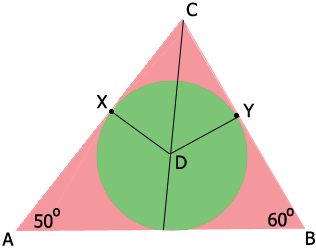SEARCH HOMEMath Central Quandaries & QueriesQuestion from Angie, a student: Circle O is inscribed in triangle ABC. Angle A = 50 and angle B = 60. Find arc XY in degreesHi Angie,

You didn't tell us what $X$ and $Y$ are. I assume they are as in my diagram where $D$ is the center of the circle and you are to find the measure of the angle $XDY.$If I am correct then I suggest that you use two facts.

1. Angles $CXD$ and $DYC$ are right angles.

2. The center of an inscribed circle in a triangle lies on the angle bisectors.

PennyMath Central is supported by the University of Regina and The Pacific Institute for the Mathematical Sciences.